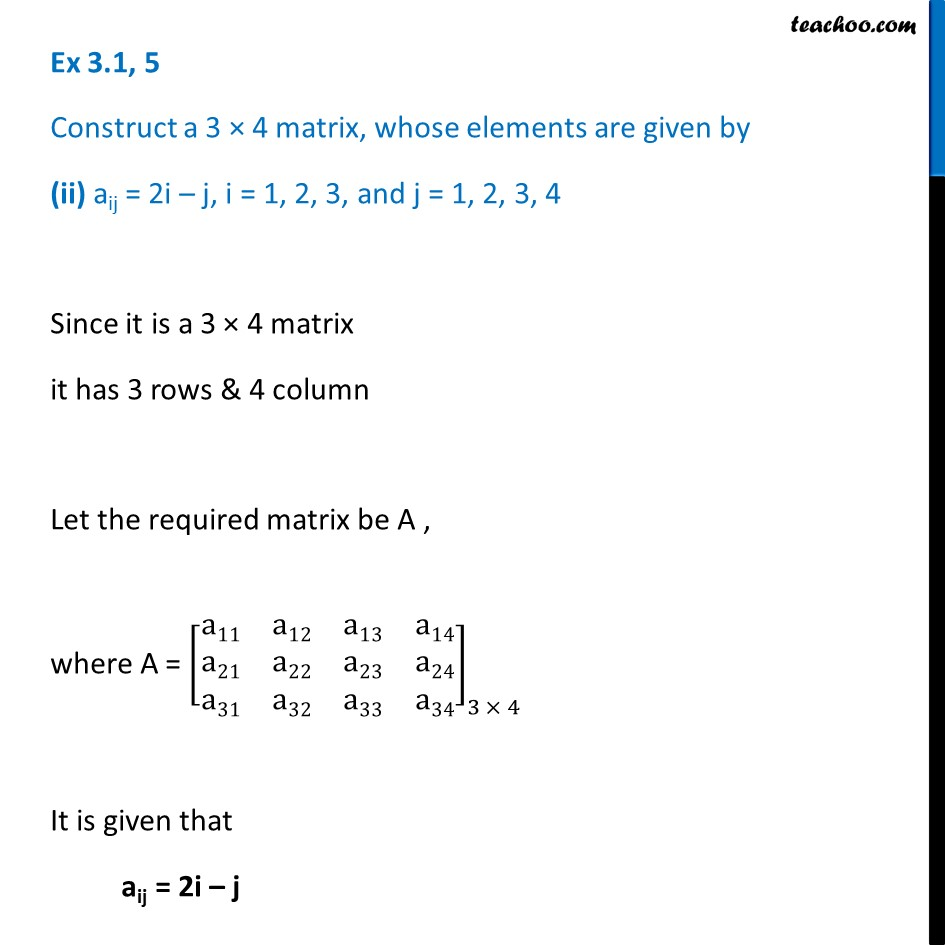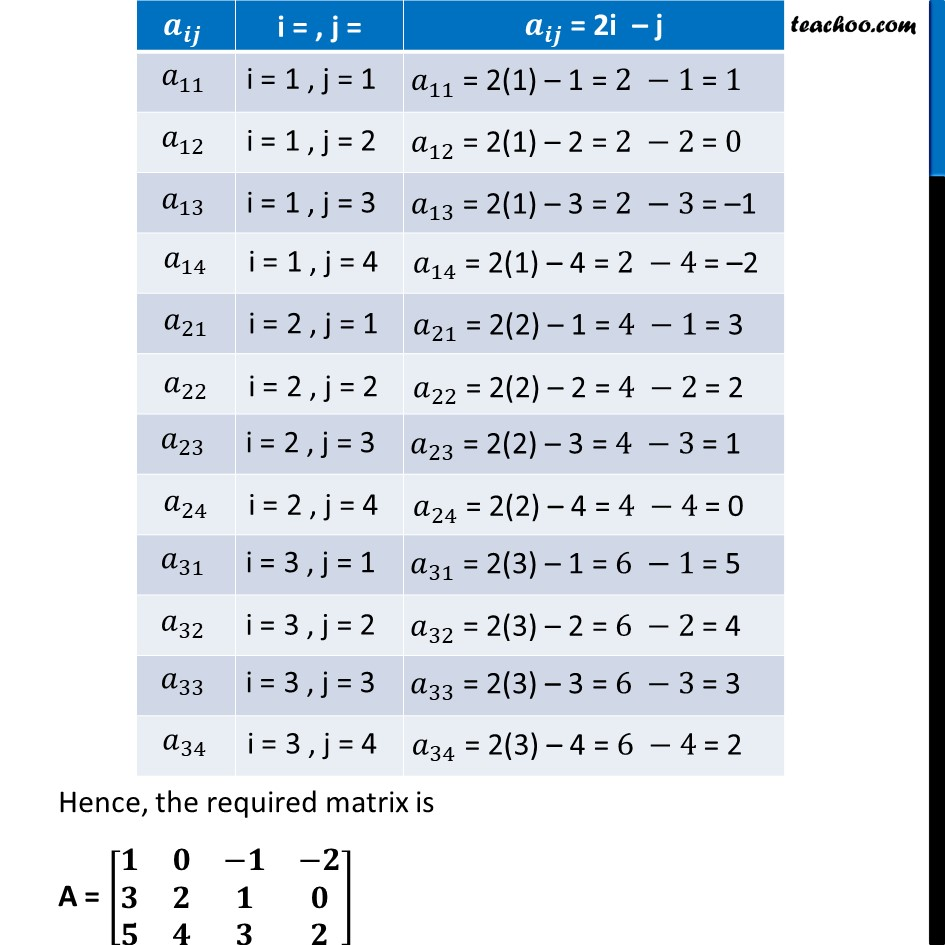Ex 3.1

Chapter 3 Class 12 Matrices
Serial order wiseLearn in your speed, with individual attention - Teachoo Maths 1-on-1 Class

### Transcript

Ex 3.1, 5 Construct a 3 × 4 matrix, whose elements are given by (ii) aij = 2i – j, i = 1, 2, 3, and j = 1, 2, 3, 4 Since it is a 3 × 4 matrix it has 3 rows & 4 column Let the required matrix be A , where A = [■8(a11&a12&a13&a14@a21&a22&a23&a24@a31&a32&a33&a34)]_(3 × 4) It is given that aij = 2i – j Hence, the required matrix is A = [■8(𝟏&𝟎&−𝟏&−𝟐@𝟑&𝟐&𝟏&𝟎@𝟓&𝟒&𝟑&𝟐)]